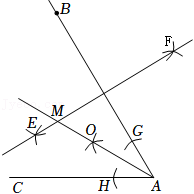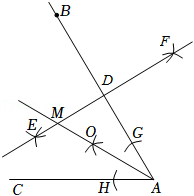(1) 分别以点 $\mathrm{A}, \mathrm{B}$ 为圆心, 大于 $\frac{1}{2} \mathrm{AB}$ 的长为半径作弧, 两弧相 交于 $\mathrm{E}, \mathrm{F}$ 两点, 作直线 $\mathrm{EF}$;
(2) 以点 $\mathrm{A}$ 为圆心, 适当长为半径画弧, 分别交 $\mathrm{AB}, \mathrm{AC}$ 于点 $\mathrm{G}$,
$\mathrm{H}$, 再分别以点 $\mathrm{G}, \mathrm{H}$ 为圆心, 大于 $\frac{1}{2} \mathrm{GH}$ 的长为半径画弧, 两弧在
$\angle B A C$ 的内部相交于点 $\mathrm{O}$, 画射线 $\mathrm{AO}$, 交直线 $\mathrm{EF}$ 于点 $\mathrm{M}$. 已知 线段 $\mathrm{AB}=6, \angle \mathrm{BAC}=60^{\circ}$, 则点 $\mathrm{M}$ 到射线 $\mathrm{AC}$ 的距离为【答案】 $\sqrt{3}$.

【解析】\begin{aligned} & \because \mathrm{AB}=6, \angle \mathrm{BAC}=60^{\circ}, \\ & \therefore \angle \mathrm{BAO}=\angle \mathrm{CAO}=\frac{1}{2} \angle \mathrm{BAC}=30^{\circ}, \quad \mathrm{AD}=\frac{1}{2} \mathrm{AB}=3, \\ & \therefore \mathrm{AM}=2 \mathrm{MD}, \end{aligned}

$$\therefore \mathrm{MD}=\sqrt{3} \text {, }$$
$\because \mathrm{AM}$ 是 $\angle \mathrm{AOB}$ 的平分线, $\mathrm{MD} \perp \mathrm{AB}$,
$\therefore$ 点 $\mathrm{M}$ 到射线 $\mathrm{AC}$ 的距离为 $\sqrt{3}$.